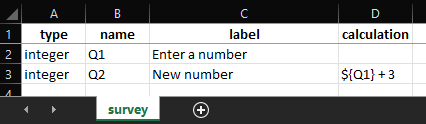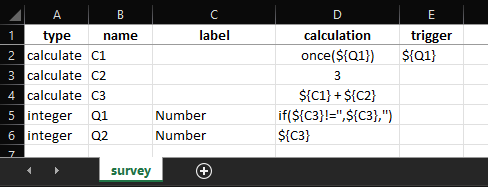# Change/ update existing variable

Hi all,

I am trying to update the value of an exiting defined variable, e.g. adding a number (e.g. 3) to an existing number.

Like when first asking a question to capture and integer “num”, then next conduct a calculation to add 3 to the variable “num” so that the new “num” = “num” + 3

Say the person enters 1 as number as asnwer, the new num variable should have the value 4.

The question is how to use an existing variable to get it updated, when I try to use the same “Data column name:” it wont accept it, maybe there is a simple other solution.

Thanks a lot.
Daan

Welcome to the community, @DaanVelthausz! Is this what you are tyring to do:

#### In the survey tab of your XLSForm:#### Reference XLSForm:

Calculation.xlsx (10.2 KB)

Dear Kam,

Thanks for the response.

It is not what I meant, I need a solution to increment a number to an existing variable, Q1 = Q1 + 3, which would look like:

integer Q1 Enter a number
integer Q1 New number \${Q1} + 3

But it does not accept to use the same variable name.

The reason I need this is to have a simple skipping check rule.
I have more than 200 selec_one questions (“Yes”/“No”) and an easy and same skipping pattern for all questions to stop with any next question after three consequative No answers. As I do not know how many questions exactly will be used, I need an easy skipping check expression.

I want to use the same integer variable to count the number of consequetaive “Yes” answers. So after each question is answered “Yes” the number should be increased with one, and after a question is answered “No” the number should be reset to zero.

Unfortnately I can not attach an excel (as new user to this community), so see here below attached as plain text what I would need but is not working as I can not use the same label name twice.

If you can not add a number to an existing variable, then the only alternative is a use for each question different skipping formula to check if the previous 3 questions were all answered yes.

Daan

type name label required relevant calculation
calculate number 0
select_one YN Q1 Question 1 \${number} <2
calculate number \${Q1}=‘1’ \${number}+1
calculate number \${Q1}=‘0’ 0
select_one YN Q2 Question 2 \${number} <2
calculate number \${Q2}=‘1’ \${number}+1
calculate number \${Q2}=‘0’ 0
select_one YN Q3 Question 3 \${number} <2
calculate number \${Q3}=‘1’ \${number}+1
calculate number \${Q3}=‘0’ 0
select_one YN Q4 Question 4 \${number} <2
calculate number \${Q4}=‘1’ \${number}+1
calculate number \${Q4}=‘0’ 0
select_one YN Q5 Question 5 \${number} <2
calculate number \${Q5}=‘1’ \${number}+1
calculate number \${Q5}=‘0’ 0
select_one YN Q6 Question 6 \${number} <2

OK maybe try this out, it should work:

#### In the survey tab of your XLSForm:#### Reference XLSForm:

Calculation.xlsx (10.4 KB)